# Magnetic field associated with a current  (Page 2/2)

 Page 2 / 2

## Case study : the right hand rule

Use the Right Hand Rule to draw in the directions of the magnetic fields for the following conductorswith the currents flowing in the directions shown by the arrows. The first problem has been completed for you.

 1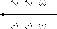2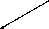3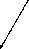45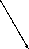6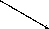78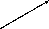9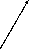1011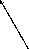12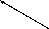## Experiment : magnetic field around a current carrying conductor

Apparatus:

1. one 9V battery with holder
2. two hookup wires with alligator clips
3. compass
4. stop watch

Method:

1. Connect your wires to the battery leaving one end of each wire unconnected so that the circuit is not closed.
2. One student should be in charge of limiting the current flow to 10 seconds. This is to preserve battery life as well as to prevent overheating of the wires and battery contacts.
3. Place the compass close to the wire.
4. Close the circuit and observe what happens to the compass.
5. Reverse the polarity of the battery and close the circuit. Observe what happens to the compass.

Conclusions:

1. Does a current flowing in a wire generate a magnetic field?
2. Is the magnetic field present when the current is not flowing?
3. Does the direction of the magnetic field produced by a current in a wire depend on the direction of the current flow?
4. How does the direction of the current affect the magnetic field?

## Case study : magnetic field around a loop of conductor

Consider two loops made from a conducting material, which carry currents (in opposite directions) and are placed in the planeof the page. By using the Right Hand Rule, draw what you think the magnetic field would look like at different points around each of the twoloops. Loop 1 has the current flowing in a counter-clockwise direction, while loop 2 has the current flowing in a clockwisedirection.

If you make a loop of current carrying conductor, then the direction of the magnetic field is obtained by applying the RightHand Rule to different points in the loop.

If we now add another loop with the current in the same direction, then the magnetic field around each loop can be added together to create a stronger magnetic field. A coil of many such loops is called a solenoid . The magnetic field pattern around a solenoid is similar to the magnetic field pattern around the bar magnet that you studied in Grade 10, which had a definite north and south pole.

## Electromagnets

An electromagnet is a piece of wire intended to generate a magnetic field with the passage of electric current through it.Though all current-carrying conductors produce magnetic fields, an electromagnet is usually constructed in such a way as to maximizethe strength of the magnetic field it produces for a special purpose. Electromagnets are commonly used in research,industry, medical, and consumer products. An example of a commonly used electromagnet is in security doors, e.g. on shop doors which open automatically.

As an electrically-controllable magnet, electromagnets form part of a wide variety of "electromechanical" devices: machines that produce a mechanical force or motion through electricalpower. Perhaps the most obvious example of such a machine is the electric motor which will be described in detail in Grade 12. Other examples of the use of electromagnets are electric bells, relays, loudspeakers and scrapyard cranes.

## Experiment : electromagnets

Aim:

A magnetic field is created when an electric current flows through a wire. A single wire does not produce a strong magnetic field,but a wire coiled around an iron core does. We will investigate this behaviour.

Apparatus:

1. a battery and holder
2. a length of wire
3. a compass
4. a few nails

Method:

1. If you have not done the previous experiment in this chapter do it now.
2. Bend the wire into a series of coils before attaching it to the battery. Observe what happens to the deflection of the needle on the compass. Has the deflection of the compass grown stronger?
3. Repeat the experiment by changing the number and size of the coils in the wire. Observe what happens to the deflection on the compass.
4. Coil the wire around an iron nail and then attach the coil to the battery. Observe what happens to the deflection of the compass needle.

Conclusions:

1. Does the number of coils affect the strength of the magnetic field?
2. Does the iron nail increase or decrease the strength of the magnetic field?

## Magnetic fields

1. Give evidence for the existence of a magnetic field near a current carrying wire.
2. Describe how you would use your right hand to determine the direction of a magnetic field around a current carrying conductor.
3. Use the Right Hand Rule to determine the direction of the magnetic field for the following situations:
4. Use the Right Hand Rule to find the direction of the magnetic fields at each of the points labelled A - H in the following diagrams.

what is kinetic friction
how to calculate the total energy needed to break all the covalent bonds in one mol of a methanol molecule?
Suggested how the difference in weight influences the size of the rocket that is needed to launch the astronaut into space from Earth
newtons third law of motion
how to deal with newtons third law of motion
Nomvulah
help me with Newtons laws of universal gravitation
Every particle in the universe attract every other particle with a force that is directly proportional to the product of their mass and inversely proportional to the square of distance between them
Delight
guys plz help me how to calculate net force?
its FN +Fg+ Ff+ Ft+Fapplied = Net Force or any force acting on that object
Thimna
FN IS A NORMAL FORCE FG IS A GRAVITATIONAL FORCE FF IS A FRICTION FORCE FA IS A APPLIED FORCE THESE ARE FORCES THAT ACT IN EVERYTHING YOU TOUCH LIKE MUG, PEN, PHONE ,MOB EVERYTHING THAT MATTER
Simphiwe
net force is all the forces acting on an Object that is your FN +FG even T +fy or fx anyforce that will act on the object
Yanga
fnet=mass×acceleration
Yanga
What's Newton second law
Mahlatse
Newton's second law...is when resultant/net force acts on an object,the object will accelerate in the direction of the force at an acceleration directly proportional to the force and inversely proportional to the mass of the object
Cheyeza
How do yu calculate normal force on a inclined
Mahlatse
net force are FORCES that ACT on that object resultant is a direction or u can say it a magnitude
Simphiwe
Newton's second law : the resultant/net force act on an object is equal to the rate of change in momentum.
Alfred
guys what is the most effective way to know the law of gravitation
Romeo
any one who can help me with chemistry
Yeah Sure. I will help
Ruthy
OK first can you pls teach me how to to calculate on chemistry chapter
george
What is a derivative?
Hulisani
Hydrocarbon derivatives are compounds that are made primarily of carbon and hydrogen atoms with specific groups of atoms attached. These specific groups of atoms are called functional groups. Hydrocarbon derivatives contain at least one element that is electronegative.
Ruthy
How to find the integral of a specific function?
Hulisani
how do u calculate the mass of an object if u have been given the gradient of a graph?
what is endothermic reaction
what is the difference between intramolecular and intermolecular forces
how to calculate atom mass
how do water molecules form
water molecules consist of two atoms of hydrogen linked by covalent bonding to the same atom of oxygen ...hydrogen bonds are formed easily when two water molecules come close together , but are easily broken when the water molecule moves apart again
Nqobile
similarity between vander waals forces and hydrogen bond
type of intermolecular forces between 2 hydrogen and 2 oxygen
Angel
when an atom X of an element in Group 1 reacts to become X+

#### Get Jobilize Job Search Mobile App in your pocket Now!By Christine ZeelieByBy Yasser IbrahimByBy Yasser IbrahimBy Jonathan LongBy Szilárd JankóBy OpenStaxBy Edward BitonBy OpenStax